Re-examination of Sales Price & Volume Variance

by P Barber

Introduction

1. This paper takes a fresh look at the process of Sales Price and Volume Variance Analysis and concludes that “Mix” provides an unsatisfactory explanation of the difference often found between High-Level and Detail-Level calculations, that this difference is driven by the combination of elements with significantly different sales prices being included within a single data set, and that the correct separation of Total Sales Variance into its Price and Volume related components is achieved by adding the detail, rather than drilling down into the data from the top level.

This process is illustrated graphically to demonstrate the issues involved. The area of the diagram below represents Sales Income in period 1.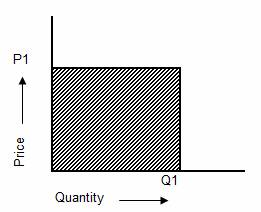Where:

P1 = Sales price-each in period 1

Q1 = Quantity sold in the period 1

The area of the shaded portion = P1 x Q1 = Sales Income in period 1 = S1

2. In the next diagram the Sales Price-each is increased from P1 to P2 and the quantity sold is increased from Q1 to Q2.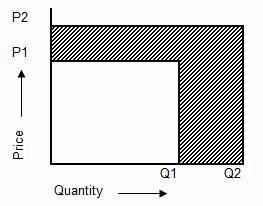Where:

P2 = Sales price-each in period 2

Q2 = Quantity sold in the period 2

S2 = Income in period 2 = P2 x Q2

3. The shaded area indicates the change in income (S2 – S1) = (P2 x Q2) – (P1 x Q1)

The challenge in Sales Price and Volume Variance Analysis is to divide this shade area into its Price related and Volume related elements.

4. The traditional set of formula (Anthony 1970) used for this application are shown below:5. However, this is not the only way in which these variances can be defined, Anthony (1970) states that the following analysis is also valid.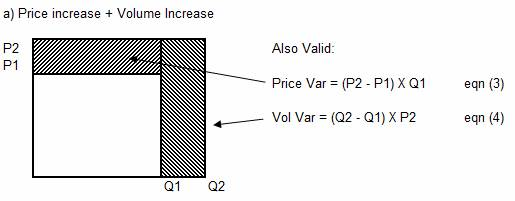6. Yet another way in which the variance can be allocated is by treating each element as a trapezium, as shown below.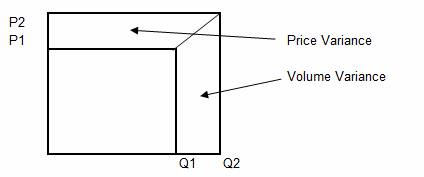7. The area of a trapezium is given by the equation:

Area of Trapezium = Width x Average Height.

Therefore the equations for Price and Volume Variance become:

Price Variance = (P2 –P1) x (Q2 + Q1) / 2                eqn (5)

Volume Variance = (Q2 – Q1) x (P2 + P1) / 2          eqn (6)

8. Each of the three sets equations (eqn 1 to 6) illustrated above are valid for situations when either both the Price and the Volume increase from period 1 to period 2, or alternatively, when both Price and Volume decrease between period 1 and period 2. The difference between the alternative equations relate to the way in which the corner (shown shaded) is divided between the two Variances.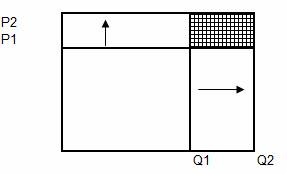9. The extreme positions are represented by the equations which either place the whole of the shaded area as Volume Variance (eqns 3 & 4), or the whole of the shaded area as Price Variance (eqns 1 & 2). There is an equal probability of the Price and Volume Variances being any value between the two extremes. If individual elements of a data-set were to be summed across the whole set then the final result would be equal to the median value between these two extremes. The geometric equations shown above (eqns 5 & 6) allocate the variance in the same way, allocating the shaded portion equally between the two extremes.

10. For convenience, in this paper, the situation where both price and volume increase between periods 1 and 2 are referred to as “type a)” while the situation where both price and quantity decrease between periods 1 and 2 are referred to as “type d)”. Equations (5) and (6) are appropriate to both of these situations.

11. The conventional formula is appropriate to situations where the price-each increases between period 1 and period 2, while the quantity is reduced. This situation, referred to in this paper as “type b)”, is illustrated below:12. The conventional formula does not however fit the situation where price-each reduces, between periods 1 and 2, while quantity increases. This situation, referred to in this paper as “type c)” is shown below: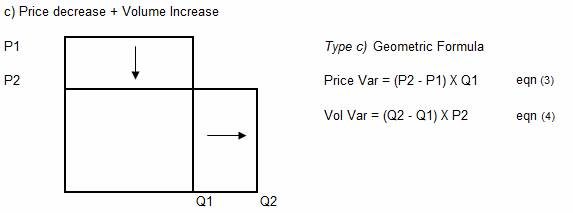13. It can be seen that the “geometry” for types b) and type c) is similar. However, parts of the equation have been swapped, with P2 replacing P1 in the Volume Variance equation and Q1 replacing Q2 in the Price Variance equation.

14. A further set of special conditions include situations where either Q1 or Q2 are equal to zero:

Type e) Q2 = 0, Q1 > 0

Type f)  Q1 = 0, Q2 > 0

Type g)  Q2 = 0, S2 <> 0

Type h)  Q1 = 0, S1 <> 0

Type e) is a discontinued product, while type f) is a new product. With both types e) and type f) all of the Sales Income change is attributable to a change in volume, the whole of the variance (S2 – S1) is therefore allocated as Volume Variance.

Types g) and type h) involve value only credit-notes and here all the Sales Income change should be allocated as Price Variance.

15. It should be noted that (Price Variance + Volume Variance) = Total Variance = (S2 – S1). This fact means that in a study of Price and Volume Variance it is only necessary to study one of the variances: either Price Variance, or Volume Variance. For practical calculation the author prefers to calculate Price Variance by backing out from the total Variance:

Price Variance = (S2 – S1 – Volume Variance )

16. The table below illustrates the Volume Variance calculated using each of the equations on a constant type a) data set.

 a) Price increase + Volume Increase Type Volume Variance Equation Q2 Q1 P2 P1 S2 S1 Vol Var a = (Q2 - Q1) X (P1 + P2) / 2 30 20 7 2 210 40 45 b = (Q2 - Q1) X P1 30 20 7 2 210 40 20 c = (Q2 - Q1) X P2 30 20 7 2 210 40 70 d = (Q2 - Q1) X (P1 + P2) / 2 30 20 7 2 210 40 45

It can be seen that the values calculated using the type a) and type d) methods (eqns 5 & 6) are identical, while the values calculated using the type b) and the type c) methods are significantly different from the values calculated using the type a)  and type d). The type b) and type c) methods show the extremes of the possible values, while type a) and type d) methods show the average of type b) and type c) methods. That is: (20 + 70)/2 = 45. It can be seen that failure to use the correct formula will result in significant analytical errors.

17. A useful insight into the way in which sales are moving is to count the number of elements which fall into each analytical type and express this information in the form shown below: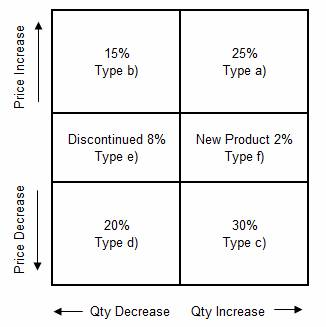Difference between Detail and High-Level Calculations

18. When performing Price and Volume Variance Analysis on a set of data it is often found that the sum of the elemental Price and Volume Variances do not add up to the values calculated at the total level. The example below, which uses the traditional equations (eqns 1 & 2) illustrates the point:

 a) Detail Calculation Set Q2i Q1i P2i P1i S2i S1i VolVari PriceVar Total A 100 80 5 3 500 240 60 200 260 B 200 40 8 6 1600 240 960 400 1360 300 120 2100 480 1020 600 1620 b) High Level Calculation Q2 Q1 P2 P1 S2 S1 VolVar PriceVar Total Total 300 120 7 4 2100 480 720 900 1620

The nomenclature used in the example above is as follows:

Q1i = Quantity sold in period 1 for each element i

Q2i = Quantity sold in period 2 for each element i

P1i = Price-each for each element in period 1. (at total level = sum(S1i)/sum(Q1i)

P2i = Price-each for each element in period 2. (at total level = sum(S2i)/sum(Q2i)

S1i = Income from each element i, in period 1 = Q1i  x  P1i

S2i = Income from each element  period 2 = Q2i  x  P2i

VolVar i = Volume Variance = (Q2i – Q2i) x P1i

PriceVar i = Price Variance = (P2i – P1i) x P2i

Q1 = S Q1i = the sum of the elemental Quantities for period 1

S = the mathematical symbol meaning the sum of all the values i = 1 to n

n = number of values in the set

Q2 = S Q2i = the sum of the elemental Quantities for period 2

S1 = S S1i = the sum of the elemental Sales Income for period 1

S2 = S S2i = the sum of the elemental Sales Income for period 2

P1 = S1 / Q1 = Average price-each for the total set in period 1

P2 = S2 / Q2 = Average price-each for the total set in period 2

VolVar  = Volume Variance = (Q2 – Q2) x P1

PriceVar  = Price Variance = (P2 – P1) x P2

In the example it can be seen that the sum of the Volume Variance in the first calculation is 1020 and the sum of the Price Variance is 600. However, the Volume Variance calculated at the Total level is 720 and the Price variance 900. In both cases the Total Variance is 1620.

The example demonstrates that the level of analysis has the effect of swapping a “piece” of the Variance between Volume and Price columns. The difference between the two calculations (VolVar: 1020 – 720 = 300) and (PriceVar: 600 – 900 = -300) has traditionally (Anthony 1970) been referred to as Mix.

This difference, also referred to as “Consolidation Adjustment” in this paper, raises a number of questions, including:

a)      What is the reason for this difference and how can it be explained?

b)      What equations can be used to explain this difference?

c)      Does this difference represent a separate Variance, or does it operate as an equal and opposite adjustment to the Price and Volume Variance total-columns?

d)      Which answer is correct, the High-Level Calculation or the sum of the Detail-Level Calculations?

e)      Can parts of this difference be assigned to individual detailed elements, or does this difference only exist at the High-Level?

f)        What steps can be taken to minimise this difference?

Can the Difference be assigned to individual detailed elements?

19. To answer this question the author conducted an experiment in which elements of data were changed and the resultant movement in Volume Variance, at the High-Level, was recorded.

It is argued that if a specific change at the detailed level results in a consistent movement in the Volume Variance, recorded at the high level, regardless of the condition of other elements in the data set, then parts of the difference can be specifically assigned to an individual detailed element.

The following assumptions were made during this experiment:

a)      That the traditional formulas for Price and Volume Variance would be used (eqns 1 & 2)

b)      That the elemental data for period 1 would remain fixed. This seemed a reasonable assumption since all the data for period 1 would be known and would not be subject to amendment as a result of information received following the end of period 2.

The initial data set for this experiment is shown below:

 Initial Data Set: Data Set Q2i Q1i P2i P1i A 100 100 8 8 B 50 50 10 10 C 200 200 12 12

While the final state of each of the data elements is given by:

 Final Data Set: Data Set Q2i Q1i P2i P1i A 80 100 10 8 B 45 50 12 10 C 180 200 14 12

The sequences in which these elements of data can be changed are set out in the table below:

 Test1 Test 2 Test 3 Test 4 A B B C A C A A

The tabular calculation below shows the Detailed and High-Level calculations for the initial state.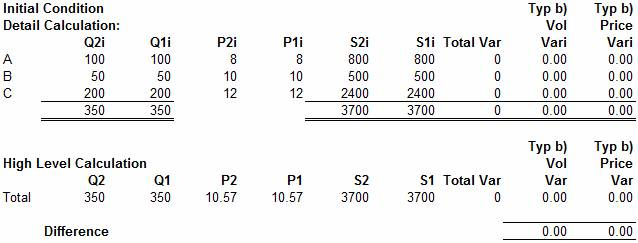With the elemental quantities: Q2i = Q1i and elemental prices: P2i = P1i, it can be seen that the elemental Volume and Price Variances equal zero:

VolVari   = (Q2i – Q1i)P1i = zero

PriceVari = (P2i –  P1i)Q2i =zero

The same result is found with the High-Level calculation, which is based on the sum of the low level quantities:

Q1 = S Q1i  and Q2 = S Q2i

and the average prices:

P1 = S (Q1i x P1i) / S Q1i  = 3700 / 350 = 10.57

P2 = S (Q2i x P2i) / S Q2i  = 3700 /350 =  10.57

With Q2 = Q1 and P2 = P1  the High-Level Price and Volume Variances are also equal to zero:

VolVar = (Q2 – Q1)P1 = zero

PriceVar= (P2 – P1)Q2 = zero

In the first test the details for element “A” are changed and the High-Level change in Price and Volume Variance is measured, between the initial state (zero as shown above) and this new result. The calculation is shown below: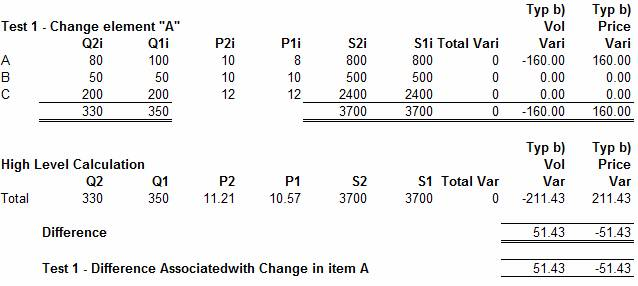Hence it can be seen that the change to the data of element “A” has caused a difference (Consolidation Adjustment) of 51.43 to the Volume Variance and -51.43 to the Price Variance Column.

In Test 2 we begin with a data set where the value of element “B” has been changed and monitor the change in “Consolidation Adjustment” when element “A” is then changed. This is shown in the calculation below: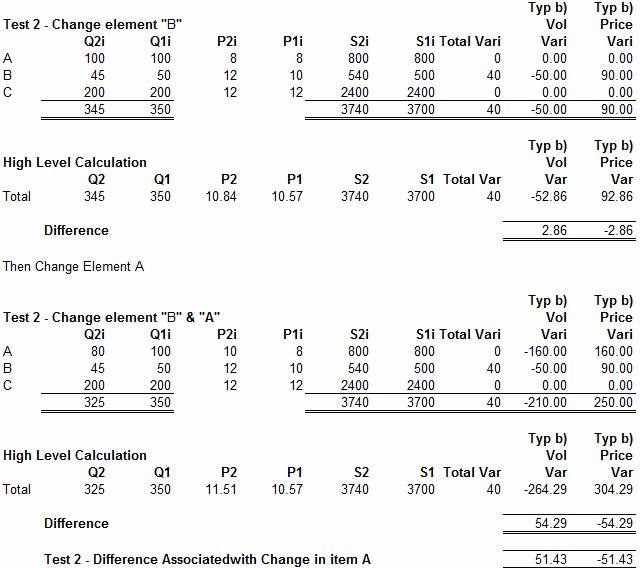As can be seen the change of 51.43 caused by the change in element “A” is the same as in the first Test. The remainder of the Tests were carried out, and in each case, [for type b) data, where price each increased between periods 1 and period 2, while the quantity sold decreased between periods 1 and period 2, using eqns (1) & (2)], the result was the same; confirming that a change in the High-Level Price and Volume Variance can be associated with a change in an individual line item. This association leads on to a consideration of the equations proposed for explaining the difference between the High Level and Detailed calculations.

20. The example above also demonstrates that the Difference between the High and Detailed-Level calculations is manifest, not as a separate variance which is measured in addition to Price and Volume Variance, but, as an equal and opposite adjustment to the Price and Volume Variance; having the effect of correcting the values calculated for both of these variances. This is a useful conclusion as it means that the same equations which account for the difference on one of the variances can also be applied to the other.

Equations defining the difference between High and Detail-level Calculations

21. Equations defining the difference between High and Detail-level calculations include:

a)      The traditional equation, described as “mix” by Anthony (1970), has the following form:

Mix = S ((Q2i – (R x Q1i)) x P1i )          eqn (7)

Where R = Q2/Q1   (the ratio of Quantity sold at the High Level, for periods 2 and 1)

b)      An alternative equation, described by the author as “level adjustment” or “the Gama adjustment” (ref 1) is as follows:

Gama  = S ((Q2i – Q1i) x (P1i – P1))          eqn (8)

c)      Another equation is:

Beta = S ((P1i – P1) x Q2i)           eqn (9)

d)      It has been known for the difference between the High and Detailed-Level calculations to be apportioned across the detailed elements using any available variable which was though to be appropriate. Such variables could include: Q1i, Q2i, S1i, S2i, VolVari, PriceVari, etc. The equation defining the elemental “Adjustment” in this case would be of the form:

Alpha(V) = S (L x vi / V)                                                 eqn (10)

Where:

L = Level Adjustment: the difference between the High and Detailed level calculations.

vi = Elemental value of the variable selected: Q1i, Q2i, VolVari, PriceVari, etc.

V= Sum of the elemental values (vi) at the High Level: Q1, Q2, VolVar, PriceVar, ETC.

It should be noted that the terms: Apha(V), Beta and Gama, have no context beyond their use in this paper, as a means of reference, and should not be confused with their application in other areas.

The difference in the results of these different calculation methods, which refers only to differences in the calculation of Volume Variance (see 20. above), is illustrated in the example below: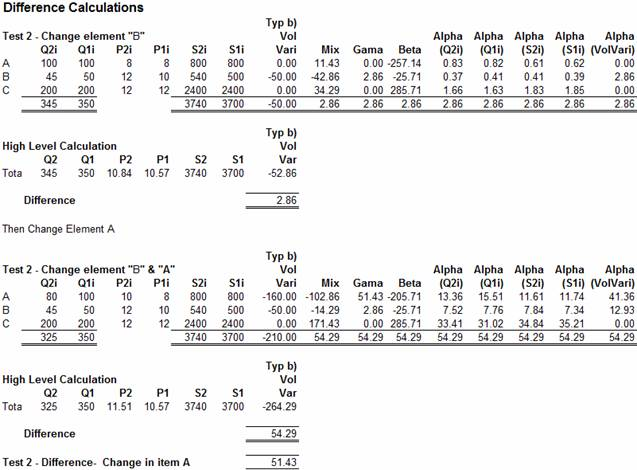The example above is based on the data used in Test2, illustrated in section 19. In examining the results shown above, first consider the first part of Test 2, which involves a change in element “B”.

Here it can be seen that the Difference between the High and Detailed Level calculations is found to be 2.86. This value is shown at the bottom of the “Typ b) Vol Vari” and is the difference between the Sum of the Volume Variances calculated at the detail level ( 0 – 50.0 – 0 = -50) and the Volume Variance calculated at the High Level (345 – 350) x 10.57 = - 52.86); with the difference being (- 50.0 - - 52.86 = 2.86)

It can be seen that each of the equations (Mix, Gama, Beta and Alph(v) ) correctly calculates the total difference. However, only the Gama and Alpa(VolVari) equations correctly associate the whole of the difference to element “B”, the only element which has changed. All the other equations spread parts of the difference across all the other elements.

Examination of the results for the second part of the test, where both elements “B” and “A” have been changed, reveals a similar pattern, here it can be seen that all of the equations correctly calculate the total Difference (-210 - - 264.29 = 54.29). However, only the Gama equation correctly states that the difference due to element “B” is 2.86 and the Difference due to element “A” is 51.43.

The Alpha(VolVari) equation no longer correctly associates the Difference to the appropriate element and all the other equations allocate the Difference in some sort of manner in which the author has struggled to provide a meaningful interpretation. Hence it is concluded that, where type b) data is involved (Price increase combined with Quantity decrease), the “Gama” equation correctly assigns the Difference to those elements which drive that Difference.

What can the Gama equation tell us about the Nature of the Difference

22. For convenience the Gama equation is reproduced below:

Gama  = S ((Q2i – Q1i) x (P1i – P1))          eqn (8)

If this equation is expanded into its component parts:

Gama = S ((Q2i – Q1i) x P1i) – S ((Q2i – Q1i) x P1)

The first term of the equation (A) is equal to the sum of the elemental Volume Variances:

(A) Sum of elemental Volume Variances = S ((Q2i – Q1i) x P1i)

While the second term (B) can be manipulated and expressed in terms of Q2, Q1 and P1 and is found to be equal to the High-Level Volume Variance:

(B) High-Level Volume Variance = S ((Q2i – Q1i) P1 = (S(Q2i) – S (Q1i))P1 = (Q2 – Q1)P1

Hence it can be seen that the two components (A) and (B) of the Gama equation merely state that the difference between the High and Detailed calculations is due to using last years’ average price as opposed to last years’ elemental price.

Difference caused by using Average price-each as opposed to Elemental price each

23. Given that the Difference is driven by the difference between the elemental and average price for the set, it can be seen that, with reference to type b) data, the Difference reduces to zero if the data set is homogeneous in regard to last years’ price each. It can be shown that this principle can be extended to cover data types a) c) and d). This important property is illustrated in the example below: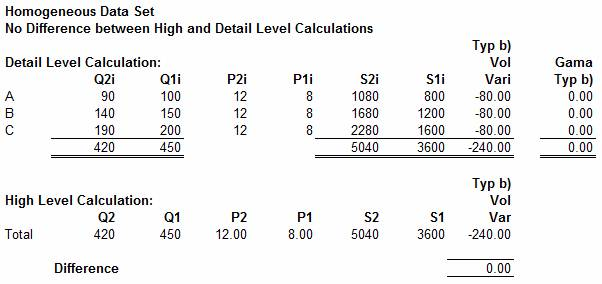As can be seen, as a result of the re-mapping, the Difference in a homogeneous data set is reduced to zero. This is a useful result as it enables the magnitude of the Difference to be reduced, by changing the counted quantity. The argument goes like this: there is nothing sacrosanct about the unit of Quantity-sold, or the price for that matter. The Sales income in periods 1 and 2 (S1i and S2i) are fixed, although there could be a change associated with the need to convert from one currency to another. If a business sold nails, it could sell them by unit, by gross (144), by 20, by 50, or by 1000, by kilo or pound (weight or sterling), the list of counting units available is endless. Since the result of a mathematical calculation should not be affected by the units of measure, it is concluded that it is valid to adjust the Quantity-sold and Price-each of elements, to establish a homogeneous set of data. The example below illustrates the principle: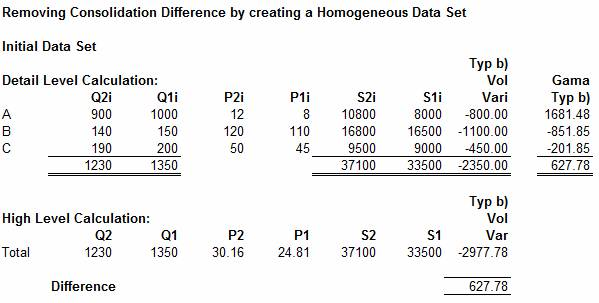Now suppose that we select a Sales price-each for period 1 of 40 units each (in practice any value could have been selected). Then we can restate the data shown above by adjusting the Quantities and Prices to reflect this change. The re-mapping process applied to element (A) is as follows:

Q2(A) = (Q2i x P1i / P1) = 900 x 8 / 40 = 180

Q1(A) = (Q1i x P1i / P1) = 1000 x 8 / 40 = 200

P2(A) = (P2i x P1 / P1i) = 12 x 40 / 8 = 60

P1(A) = (P1i x P1 / P1i) = 8 x 40 / 8 = 40 = P1

Where P1 = the value selected for the Price-each in period 1, in this case 40 units each.

The other elements (B) and (C) are remapped in the same way to establish the data set shown below:As can be seen, the effect of calculating the Volume Variance from the re-mapped data is to provide a calculation at the High-Level which is free from “Consolidation Error”.

An important conclusion to be drawn from this analysis is that the correct separation of the Total Sales Variance into its Volume and Price related component parts can be achieved by summing up the detailed elemental analysis.

Top-down or Bottom-up

24. Anthony (1970) regards the Difference between High and Detailed-Level calculations to be due to “Mix” and states that “the mix phenomenon arises whenever a cost or revenue is analysed by component, rather than in total. If revenue is analysed by individual products, or by individual geographic regions, a mix variance inevitably arises. Failure to appreciate this fact can lead to great frustration in trying to make the figures add-up properly.”

Anthony sees variance analysis as a tops-down process, where the Analyst identifies a significant variance at the top-level, and then drills-down into the data to determine the reason for the variance. Anthony implies that the deeper the analyst drills the data the greater the amount of “mix” becomes.

Another feature of drilling data is that the data is divided into smaller and smaller elements. There comes a point where it is no longer possible to analyse the data, because time, product and customer comparisons are no longer available, and it is therefore not possible to create meaningful elemental sets as more data becomes classified as either a new product: type f), or a discontinued product: type f) and more and more of the data is classified as Volume Variance.  Given the apparent futility of drilling further and further into the data, in an attempt to find “the correct answer” it is temping to regard the answer calculated at the High-Level to be the “correct answer”, and “Mix” to be an error, introduced by the Analyst as a result of drilling the data.

25. However, the analysis above demonstrates that the Difference between High and Detailed-Level calculations is caused by combining data elements which have significantly different Sales prices. And it is the significantly different Sales prices which cause a “Consolidation Error” to be created when calculations are carried out at the High-Level. It is therefore concluded that a correct separation of  Sales Variance into its respective Price and Volume Variance components is best achieved by calculating from  price-homogeneous elements and summing the elemental variances to create the High-Level Variances. However, in practice it is found that it is often not possible to fully eliminate the effects of “Consoildation Error” and it is therefore recommended that the “Gama equation(s)” be used to identify the amount of error associated with individual elements of the data set.

It should be noted that the original application of “Mix” (Anthony 1970) was to materials consumption, where variances were measured against a standard. Mix Variance applied to situations where the proportion of raw materials deviated from standard. As such a separate and independent “Mix Variance” was created. “Sales Income Volume and Price Variance Analysis” does not involve the use of a Standard and the use of the traditional “Mix” equation does not appear to be appropriate to this application.

Data Analysis involving all Data Types

The above analysis concentrated on type b) data, where price each increases and quantity decreases between periods 1 and 2. The full set of equations for each data type are shown below: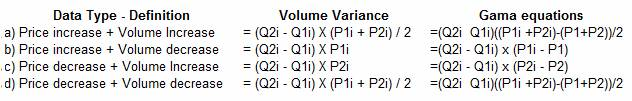Where:

Price Variance = (Q2i x P2i) – (Q1i x P1i) – Volume Variance(i)

Q1i = elemental quantity sold in period 1

Q2i = elemental quantity sold in period 2

P1i = elemental price-each in period 1

P2i = elemental price-each in period 2

P1 = Average Price for the whole set in period 1

P2 = Average Price for the whole set  in period 2

These equations are used in the example below which incorporates all the main data types in a single calculation:In the above example the total quantities and average prices for the whole set are assessed and at the High-Level the set is found to be of type d). The calculation of Volume variance (VolVar) is then carried out using the equation appropriate to the elemental data type with the results shown in the column headed “Low Level VolVar”. The Sum of the elemental Volume Variances is equal to

minus 1630, while the Volume Variance calculated at the High-Level is equal to minus 2247.5. The difference between these two numbers, the Consolidation Error, is equal to minus 617.52.

The next stage in the calculation is to calculate the elemental Volume Variance using the equation appropriate to the total set, in this case using the equations for type d), since this was found to be the type for the total set. These calculations are shown in the column headed “ Vol Var Calc as Type d)”, the sum of this column is minus 2260

The difference between the two elemental Volume Variance calculations is shown in the column headed “Diff due to Type”. The total of the column is equal to minus 630 (2260 - 1630)

The next column shows the Gama Adjustment appropriate to the High-Level data type, in this case type d), and the sum of the column is equal to 12.48

The final column shows the sum of the “Diff due to Type” and the “Gama Adjustment”, to reveal the elemental “Total Consolidation Error”. The sum of the “Total Consolidation Error” is equal to minus 617.52 (12.48 – 630), the same value as shown at the bottom of the calculation, as the difference between the High and Detail-Level calculations.

It should be noted that correct value for the Volume Variance at the High-Level is minus 1630 and the correct Price Variance at the High-Level is minus 1085 (the Total VarianceVolume Variance = (-2715 - - 1630) = 1085). The “Consolidation Error” is minus 617.52 and this is relevant when trying to explain why the “High-Level” calculations of Price and Volume Variance was not correct.

The author spent fourteen years in a senior position with a large lighting manufacturer. He had Pan-European responsible for Sales Analysis down to Gross Margin level, before leaving this position in May 2001, to pursue other career opportunities. During the following six years he continued  to investigate the nature of the Difference between the High and Detailed-Levels of Price and Volume Variance Analysis.

References

Ref (1) The Author developed the type b) Gama equation from first principles during his period in the lighting industry, where the equation was incorporated in the “Gama Analytical System”. The subsequent Gama equations were developed after leaving the lighting industry. However, it is possible that the type b) Gama equations were developed by other researchers prior to their development and usage by the Author.

Anthony, Robert N. 1970. Management Accounting: Text and Cases, Richard D. Irwin, Inc.